# Getting started with MATLAB: A Quick Introduction

Published  February 24, 2018   0MATLAB (Matrix Laboratory) is a programming platform developed by MathWorks, which uses it's proprietary MATLAB programming language. The MATLAB programming language is a matrix-based language which allows matrix manipulations, plotting of functions and data, implementation of algorithms, creation of user interfaces, and interfacing with programs written in other languages, including C, C++, C#, Java, Fortran and Python. It is used in a wide range of application domains from Embedded Systems to AI, mainly to analyze data, develop algorithms, and create models and applications.

### MATLAB Main Window

When you launch MATLAB software, MATLAB desktop appears containing tools, variables and application related with MATLAB. The Desktop will somehow look like the image below. You can even customize the arrangement of tools and documents as per your need. The three main parts appears on the screen are Command Window, Workspace and History.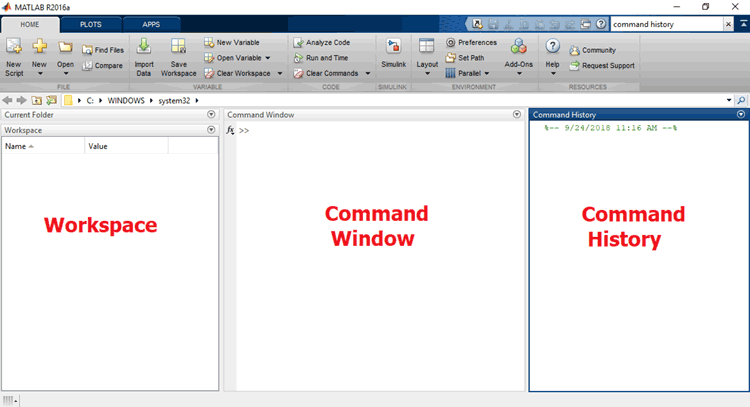### Desktop tools of MATLAB

The desktop tools of MATLAB are Command Window, Command History, Work space, Editor, Help, Array Editor, and Current Directory Browser. Here we will explain all the tools one by one.

### 1. Command Window

Command window is used to entering variables and to run a function and M-file scripts. Up (↑) Arrow key is used for recalling a statement which is previously entered. After recalling you can edit that function and press enter to run it.

Some basic operation which can be performed on command window:

For creating a row vector with any number 1, 2, 3, 4, 5 and assigning it to variable ‘x’,

``` » x = [1, 2, 3, 4, 5]
x =

1   2   3   4   5```

To create the column vector with the number 6, 7, 8, 9, and assigning it to variable ‘y’,

``` » y = [6; 7; 8; 9]
y =

6
7
8
9```

Also we can create a column vector by the help of a row vector (property of matrix),

``` » y = [6 7 8 9]
y =

6   7   8   9

» y'
ans =

6
7
8
9```

If we want to make a row vector from 0, 1, 2, 3, 4, 5, 6, 7, 8 then we can simply write as

```» a = [0:8]
a =

0   1   2   3   4   5   6   7   8```

If we want to make a row vector with increment by 2 then simply write

```» u = [0:2:8]
u =

0   2   4   6   8```

And for decrement by 2

```» u = [12:-2:0]
u =

12   10   8   6   4   2```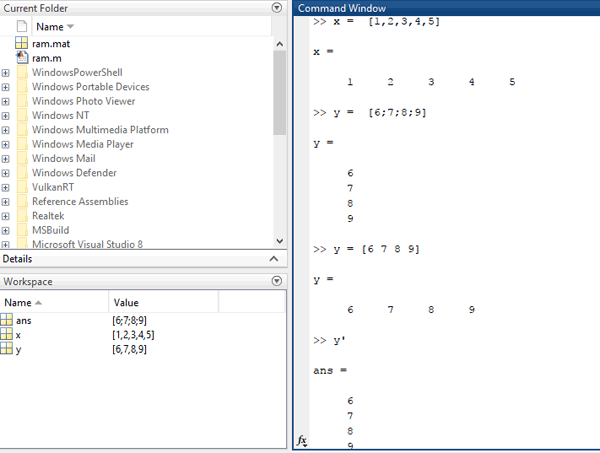Now, for performing simple mathematical operation like addition and subtraction, lets take any two numbers 12 and 14.

```» u = 12+14
ans =

26```

For subtraction

```» u = 12-14
ans =

-2```

### 2. Command History

Command history means the history of the command window.

It means the function or lines you entered in the Command window is also visible in the Command History window. Even we can select any previously entered function or line and execute it. Also, you can create an M-file for selected statement. M-File is nothing but a text file which contains MATLAB code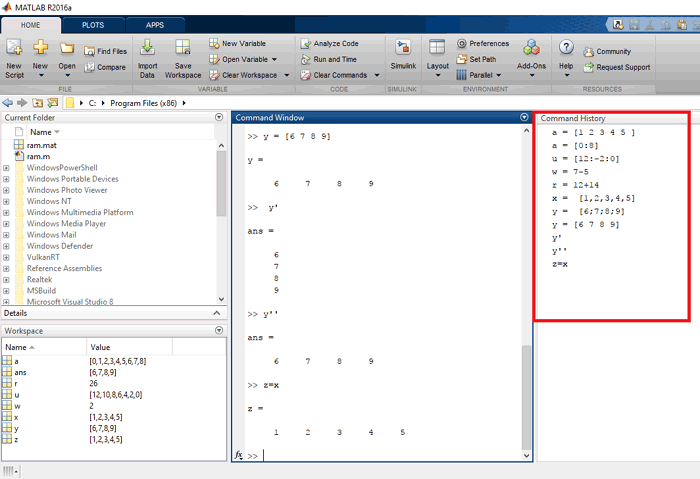### 3. Workspace

MATLAB workspace consists of set of variables made during the time of performing mathematical operations, running saved M-files, and loading saved workspaces. For deleting variables from the workspace, select any particular variable click on EDIT then DELETE. As you exit from MATLAB, it automatically clears the workspace. For saving it for later MATLAB session, click on Workspace Action Icon then SAVE, this will save your workspace into a MAT-file, with an extension of “.mat”. For reading it in next session you have to import that file, by clicking on FILE then IMPORT DATA.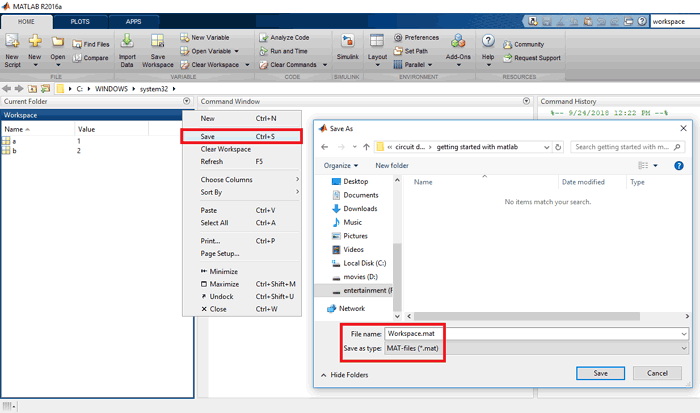### 4. Editor Window

Editor is a word processor specifically designed for creating and debugging M-files. An M-file consists of one or more commands to execute. After saving the M-file, you can even call it directly by typing the file name in command history.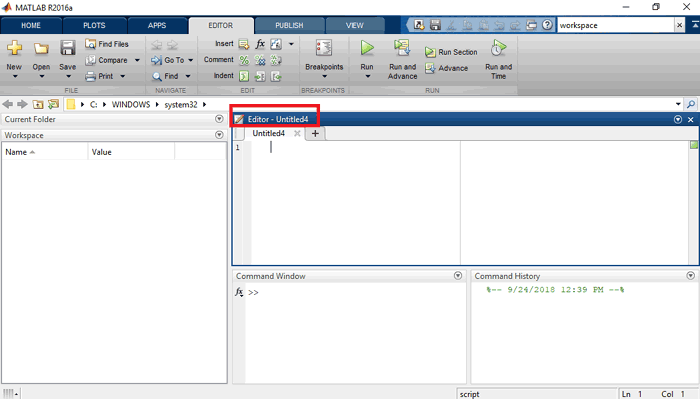### 5. HELP

To open the Help browser, click on the HELP button in the MATLAB desktop tools or alternate for HELP browser is to go to command window and type help browser. Use HELP browser for the finding the information, indexing, searching and Demos. While reading the documentation, you can bookmark any page, print a page, search for any term in the page and copy or evaluate a selection.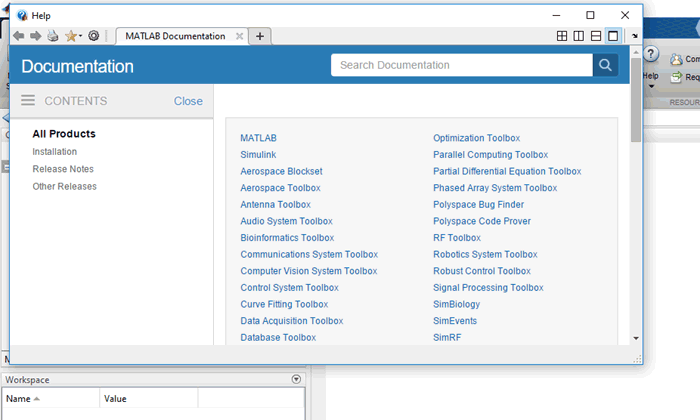### 6. Array Editor

In the Workspace Browser double click on a variable to see it in the Array Editor. Array editor is used for viewing and editing a visual representation of variables in workspace.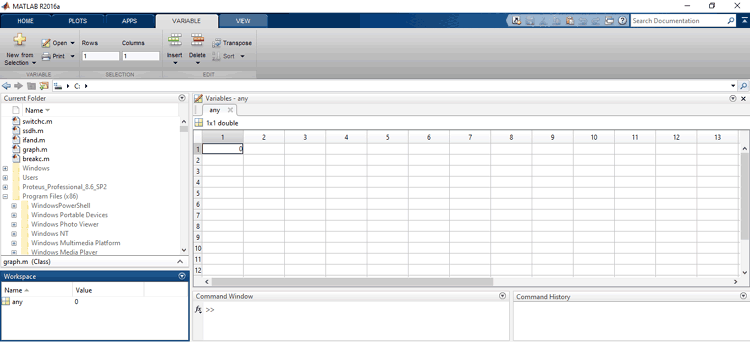### 7. Current Directory Browser

MATLAB file operations use search path and current directory as reference point. A quick way to browse your MATLAB file is using Current Directory Browser. We can use Current Directory Browser for searching, viewing and editing of the M file or MATLAB file.

Now if we save more than two files, in which one is for plotting of graph and the other one is for matrix manipulation in MATLAB file so we can access these saved files by using command window.

### Variable in MATLAB

There is no need of any type of declaration or dimension statements in MATLAB. When we construct a new variable name in MATLAB, it automatically creates the variable and provides the appropriate amount of storage and save in workspace. If the variable with the same name is already present, MATLAB changes its contents and allocate new storage if required. Variable name consists of letter and followed by letters, digits or underscore. Also, MATLAB is case sensitive it distinguish between lower and upper case.

For example:

```» x = 0
x =

0

» y = 1
y =

1```

We can also create the vector with help of simple variable like this

```» x = [0 1 2 3 4 5 6]
x =

0   1   2   3   4   5   6```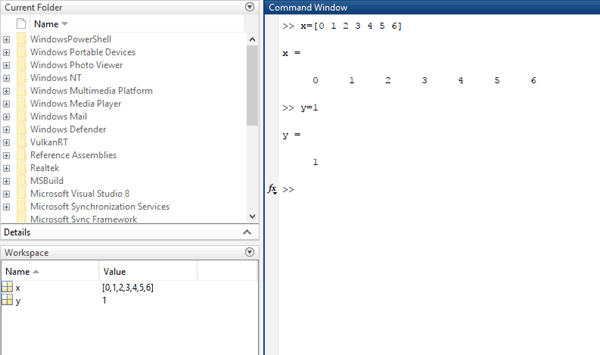### M-Files

M-files are text file contains MATLAB code created by the user. For creating M-file you can use MATLAB EDITOR or another text editor. M-files are saved with extension “.m”. For example:

`» A = [2 4 5 6 7]`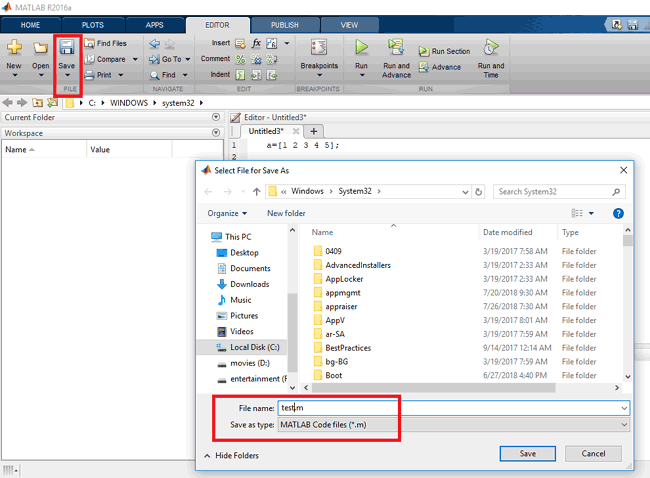Store the file under the name test.m, then the statement test in the command window reads the file and creates a variable A, which contains our matrix or the data saved in that M-file.

### Graph Plotting

MATLAB has facilities to display the vector and matrix in the form of graph, depending on the type of input data.

For example: Plot a graph between the ‘x’ and ‘y’.

Let the range of the ‘x’ is 0 (zero) to π (pi) and the ‘y’ is the sine function of ‘x’ with range of 0 to π (pi)

```» x = 0:pi/5: pi;
» y = sin(x);```

below command is used for plotting graph in between  x and y

`» plot(x,y);`

For labelling x and y axis

```» xlabel('range of y');
» ylabel('sin of x');```

And the title of the graph given as

`» title('plot of sin(x)');`

Result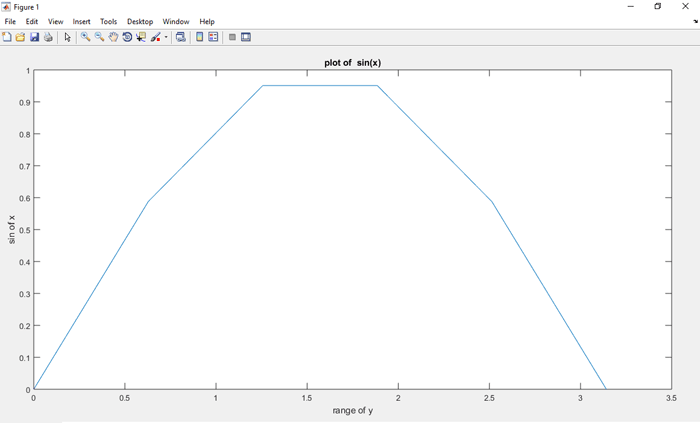Another example of the plotting of curve

Let two variables be x, y for plotting the simple y=x straight line,

```» x= 0:2:20;
» y = x;
» plot(x,y);
» xlabel('X');
» ylabel('Y');
» title('plot of the y=x straight line');```

Result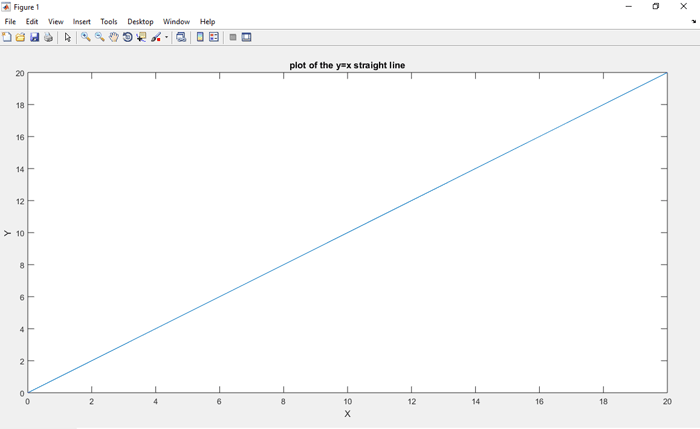We can also plot the graph of the any trigonometric function, algebraic function and the graph of matrices manipulation.

### Condition Statements in MATLAB

Like we use condition statement in various software’s while programming our microcontrollers, we can also use them in MATLAB programming. The several condition statements used in MATLAB are:

• for loop
• while loop
• if statement
• continue statement
• break statement
• switch statement

### If statement

For evaluating a logical expression and executes a group of statements only when the condition is true, ‘if’ statement is used. ‘elseif’ and ‘else’ are used for execution of alternative groups of statements.

```» if a > b
fprintf (‘greater);
elseif a == b
fprintf( ‘equal’ );
elseif a < b
fprintf (‘less’);
Else
fprintf(‘ error’);
end
```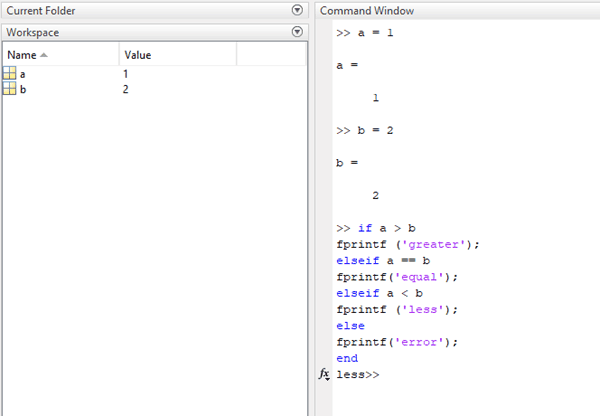### Switch and Case Statement

In the switch statement the group of statement execute based on the value of variable or expression.

Example:

```» x = input(‘Enter the no: ‘);

switch x
case 1
disp('the number is negative')
case 2
disp('zero')
case 3
disp('the number is positive')
otherwise
disp('other value')
end     ```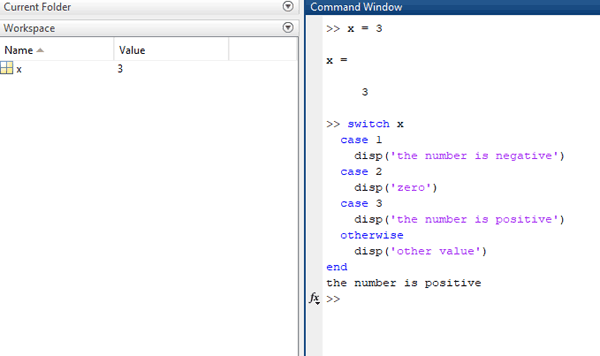### Break Statement

Break statement is used for exit from a while loop or for loop early. While it breaks from the innermost loop only in nested loops.

Example:

```» x = 2;
while (x < 12)
fprintf('value of x: %d\n', x);
x = x+1;
if(‘ x > 7’)
break;
end
end```

After the execution of code the result will be:

```value of x: 2
value of x: 3
value of x: 4
value of x: 5
value of x: 6
value of x: 7```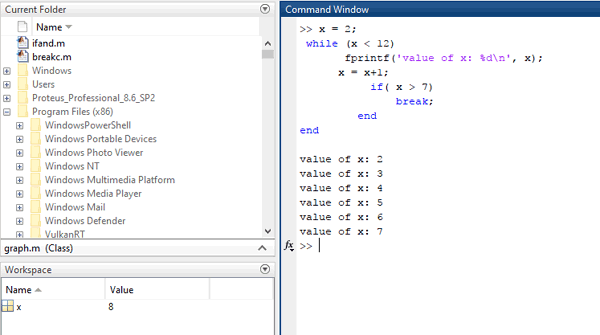### Continue statement

This statement used inside the loops. The control jumps to the start of the loop for next iteration, by skipping the execution of the statement inside the body of the current iteration of the program.

Example:

```» x = 2;

while(x<12)
if x == 7
x = x+1;
continue;

end
fprintf('value of x: %d\n', x);
x = x+1;
end```

Hence, the result will be:

```value of x: 2
value of x: 3
value of x: 4
value of x: 5
value of x: 6
value of x: 8
value of x: 9
value of x: 10
value of x: 11```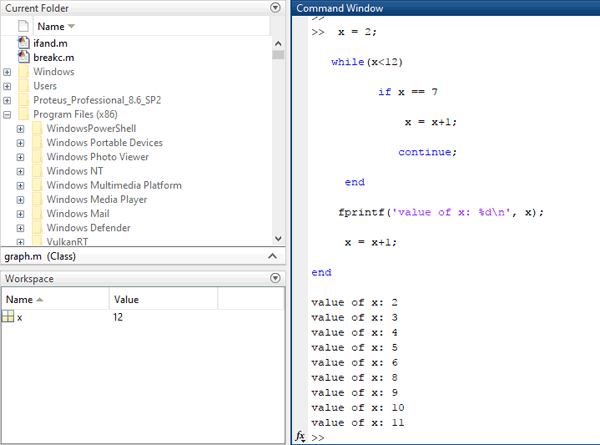### For loop

The FOR loop repeats a group of statement in fixed no. of times. The syntax of the FOR loop is as following:-

```for <index = value>
……………….
end```

Example:

```» for x = [2, 1, 3, 4, 5]
disp (x)
end
2

1

3

4

5```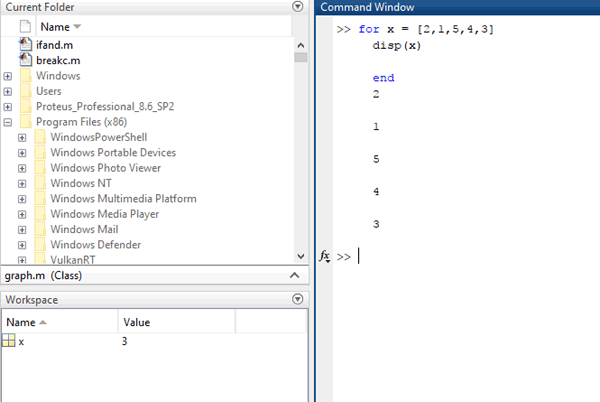### While loop

When a specified condition is true the while loop is repeatedly execute the statement

The syntax of a while loop is as following:-

```while <expression>
<statement>
end```

Example:

```» x = 2;
while( x< 18 )
fprintf('value of x: %d\n', x);
x = x + 1;
end```

The result of this loop when code is executed

```value of x: 2
value of x: 3
value of x: 4
value of x: 5
value of x: 6
value of x: 7
value of x: 8
value of x: 9
value of x: 10
value of x: 11
value of x: 12
value of x: 13
value of x: 14
value of x: 15
value of x: 16
value of x: 17```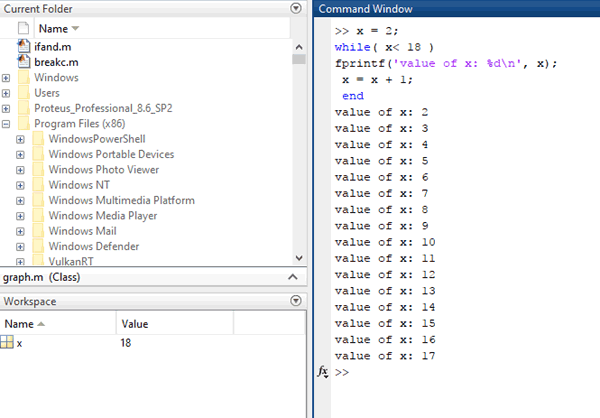This is just a introduction of MATLAB, it has very vast and complex applications. A beginner can start MATLAB with below basic projects: